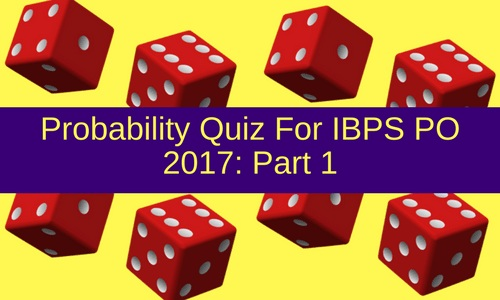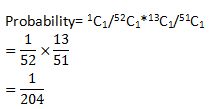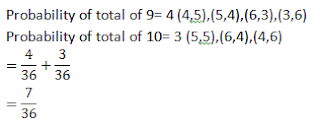# Probability Quiz For IBPS PO 2017: Part 1Q.1 From a pack of 52 cards two cards are drawn at random one after another, what is the probability that the first card is king of spade and another card is diamond?
A. 1/52
B. 1/204
C. 13/52
D. 1/208
E. None of these

Q.2 In a simultaneous thrown of two dice. What is the probability of getting a total of either 9 or 10?
A. 1/6
B. 5/36
C. 7/36
D. 1/18
E. None of these

Q.3 In a beg there are 5 blue, 3 green and 7 pink balls. 2 balls are drawn at random. What is probability thal one is blue and second is pink?
A. 1/10
B. 2/15
C. 1/6
D. 1/3
E. None of these

Q.4 4 apples and 5 mangoes are kept in a box. If 3 fruits are choosen at random, What is the probability that at least one is mango?
A. 1/8
B. 5/6
C. 20/21
D. 4/9
E. None of these

Q.5 What is the probability of getting the total of at least 8 when two dice are thrown?
A. 5/18
B. 2/5
C. 1/6
D. 5/12
E. None of these

#### 1. Ans. B.

Solution:#### 2. Ans. C.

Solution:#### 3. Ans. D.

Solution:#### 4. Ans. C.

Solution:#### 5.  Ans. D.

Solution: Probability of total of 8= 5 (5,3),(4,4),(3,5),(6,2),(2,6)
Probability of total of 9= 4 (4,5),(5,4),(6,3),(3,6)
Probability of total of 10= 3 (5,5),(6,4),(4,6)
Probability of total of 11= 2 (5,6),(6,5)
Probability of total of 12= 1 (6,6)
=5/36+4/36+3/36+2/36+1/36
=5/12